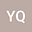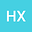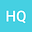Numerical simulation of a two compartmental fractional model in pharmacokinetics and parameters estimation
•••• Yanli Qiao,
• Huanying Xu,
• Haitao Qi
Yanli Qiao
Shandong University

Corresponding Author:[email protected]

Author ProfileHuanying Xu
Shandong University
Author ProfileHaitao Qi
Shandong University
Author Profile## Abstract

Compartmental model is the classic and widely used approach in pharmacokinetics. Recently, fractional calculus is introduced to describe the time course of drugs in human body which follow the anomalous diffusion mechanism. To consider the different fractional order transmission process, the two compartmental fractional model has been proposed and studied. And, it will be of great significance to find out a simple and efficient numerical method to solve the model and estimate model parameters. This work investigates two numerical methods of the two compartmental fractional model using finite difference schemes, which are based on the shifted Grünwald-Letnikov approximate formula and L1 formula, respectively. The hybrid Nelder-Mead simplex search and particle swarm optimization, denoted as NMSS-PSO, is provided to estimate the fractional model parameters. Comparison between the numerical solution and the solution by the numerical inverse Laplace transform method establishes the validity of the developed numerical algorithms. Then, the influence of the order of fractional derivative on the drug amount in human body is also discussed. Finally, the two compartmental fractional model is applied to fit the amiodarone pharmacokinetics data based on the NMSS-PSO algorithms. This work will be of importance for the development of fractional pharmacokinetics.
22 Apr 2020Submitted to Mathematical Methods in the Applied Sciences
29 Apr 2020Submission Checks Completed
29 Apr 2020Assigned to Editor
01 May 2020Reviewer(s) Assigned
14 Apr 2021Review(s) Completed, Editorial Evaluation Pending
14 Apr 2021Editorial Decision: Revise Major